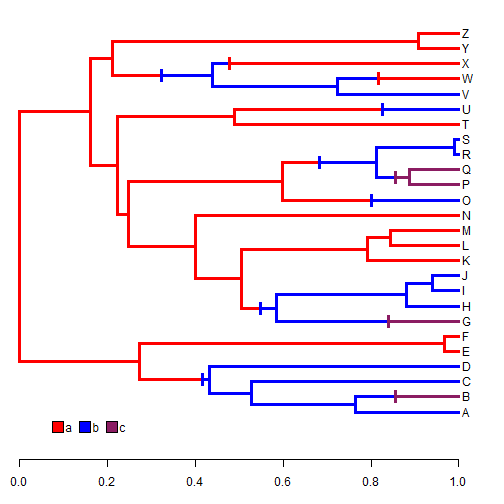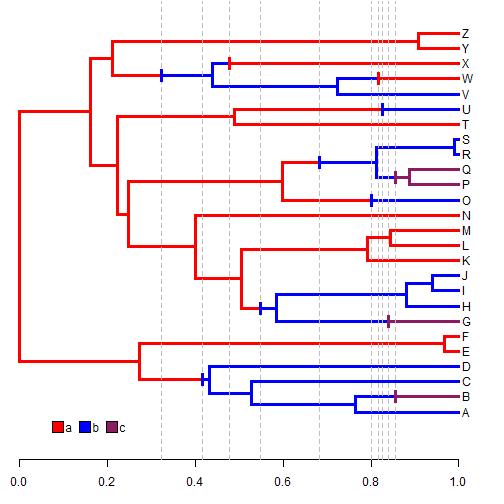## Saturday, August 8, 2015

### Demarcating character changes on a tree with stochastically mapped discrete character

In the process of working on another problem (actually, answering a phytools user question), I happened upon the problem of marking changes on a plotted tree with mapped discrete character.

I decided to try to write a function that does this, and the following is the result. Note that this has barely been debugged, so it is offered without guarantees:

``````markChanges<-function(tree,colors=NULL,cex=1,lwd=2){
states<-sort(unique(getStates(tree)))
if(is.null(colors)) colors<-setNames(palette()[1:length(states)],
states)
obj<-get("last_plot.phylo",envir=.PlotPhyloEnv)
nc<-sapply(tree\$maps,length)-1
ii<-which(nc>0)
nc<-nc[ii]
h<-vector()
for(i in 1:length(ii)){
for(j in 1:nc[i]){
ss<-names(tree\$maps[[ii[i]]])[j+1]
mm<-tree\$edge[ii[i],1]
dd<-tree\$edge[ii[i],2]
x<-rep(obj\$xx[mm]+cumsum(tree\$maps[[ii[i]]])[j],2)
y<-c(obj\$yy[dd]-0.5*mean(strheight(LETTERS)*cex),
obj\$yy[dd]+0.5*mean(strheight(LETTERS)*cex))
lines(x,y,lwd=lwd,col=colors[ss],lend=2)
h<-c(h,x)
}
}
invisible(h)
}
``````

Let's try it with a simulated tree:

``````library(phytools)
Q<-matrix(c(-1,1,0,1,-2,1,0,1,-1),3,3)
colnames(Q)<-rownames(Q)<-letters[1:3]
Q
``````
``````##    a  b  c
## a -1  1  0
## b  1 -2  1
## c  0  1 -1
``````
``````tree<-sim.history(pbtree(n=26,tip.label=LETTERS,scale=1),
Q,anc="a")
``````
``````## Done simulation(s).
``````
``````colors<-setNames(c("red","blue","maroon4"),
sort(unique(getStates(tree,"tips"))))
colors
``````
``````##         a         b         c
##     "red"    "blue" "maroon4"
``````
``````plotSimmap(tree,colors,ylim=c(-1,Ntip(tree)+1),lwd=3,
mar=c(3.1,0.1,0.1,0.1))
markChanges(tree,colors,lwd=3)
y=0,vertical=FALSE,prompt=FALSE)
axis(1)
``````You may have noticed that the function (invisibly) returns a vector containing the heights above the root of all changes. This enables us to do things like the following:

``````plotSimmap(tree,colors,ylim=c(-1,Ntip(tree)+1),lwd=3,
mar=c(3.1,0.1,0.1,0.1))
xx<-markChanges(tree,colors,lwd=3)
axis(1)
obj<-sapply(xx,function(x) lines(rep(x,2),par()\$usr[3:4],
lty="dashed",col="grey"))